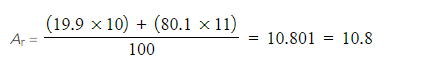# AQA A Level Chemistry复习笔记1.1.2 Mass Number & Isotopes

### Subatomic Structure of Atoms & Ions

• The mass of an atom is concentrated in the nucleus, because the nucleus contains the heaviest subatomic particles (the neutrons and protons)
• The mass of the electron is negligible

• The nucleus is also positively charged due to the protons
• Electrons orbit the nucleus of the atom, contributing very little to its overall mass, but creating a ‘cloud’ of negative charge
• The electrostatic attraction between the positive nucleus and negatively charged electrons orbiting around it is what holds an atom together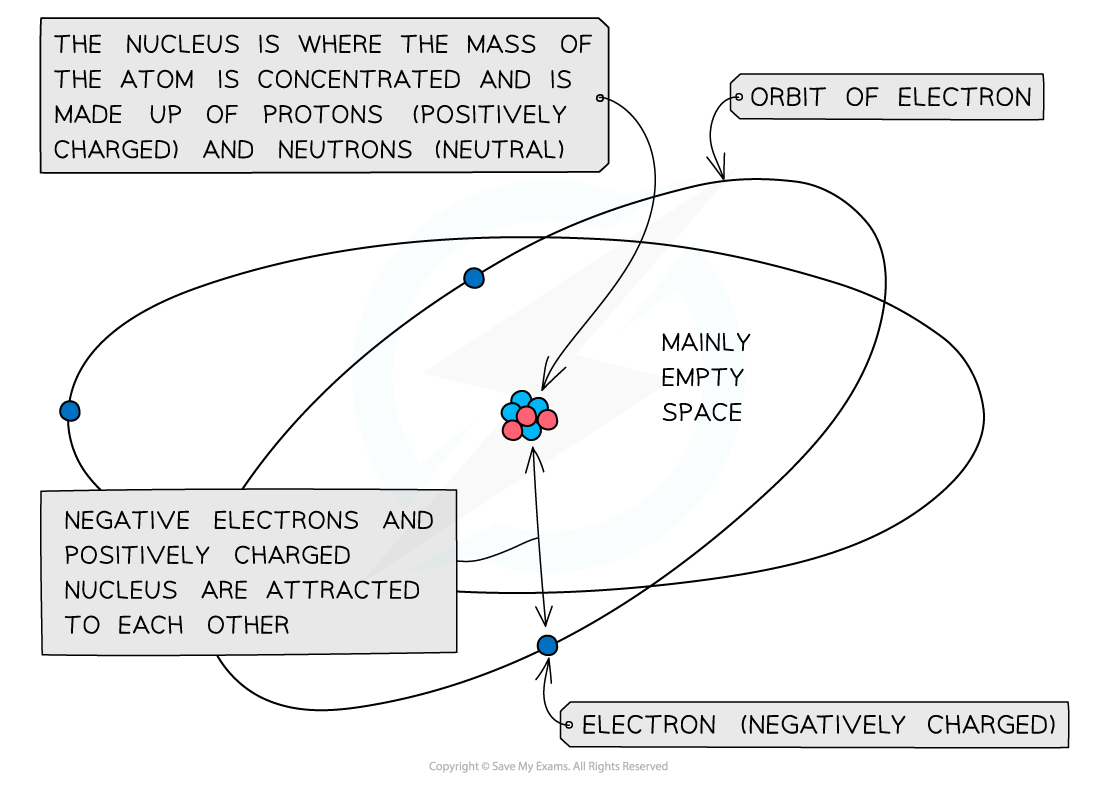The mass of the atom is concentrated in the positively charged nucleus which is attracted to the negatively charged electrons orbiting around it

• An atom is neutral and has no overall charge
• Ions on the other hand are formed when atoms either gain or lose electrons, causing them to become charged
• The number of subatomic particles in atoms and ions can be determined given their atomic (proton) number, mass (nucleon) number and charge

#### Protons

• The atomic number of an atom and ion determines which element it is
• Therefore, all atoms and ions of the same element have the same number of protons (atomic number) in the nucleus
• E.g. lithium has an atomic number of 3 (three protons) whereas beryllium has atomic number of 4 (4 protons)

• The number of protons equals the atomic (proton) number
• The number of protons of an unknown element can be calculated by using its mass number and number of neutrons:

Mass number = number of protons + number of neutrons

Number of protons = mass number - number of neutrons

#### Worked Example

Determine the number of protons of the following ions and atoms:

1.  Mg2+ ion
2.  Carbon atom
3.  An unknown atom of element X with mass number 63 and 34 neutrons

Answer 1: The atomic number of a magnesium atom is 12 indicating that the number of protons in the magnesium element is 12

• Therefore the number of protons in a Mg2+ ion is also 12

Answer 2: The atomic number of a carbon atom is 6 indicating that a carbon atom has 6 protons in its nucleus

Answer 3: Use the formula to calculate the number of protons

Number of protons = mass number - number of neutrons

Number of protons = 63 - 34

Number of protons = 29

• Element X is therefore copper

#### Electrons

• An atom is neutral and therefore has the same number of protons and electrons
• Ions have a different number of electrons to their atomic number depending on their charge
• A positively charged ion has lost electrons and therefore has fewer electrons than protons
• A negatively charged ion has gained electrons and therefore has more electrons than protons

#### Worked Example

Determine the number of electrons of the following ions and atoms:

1. Mg2+ ion
2. Carbon atom
3. An unknown atom of element X with mass number 63 and 34 neutrons

Answer 1: The atomic number of a magnesium atom is 12 suggesting that the number of protons in the neutral magnesium atom is 12

• However, the 2+ charge in Mg2+ ion suggests it has lost two electrons
• It only has 10 electrons left now

Answer 2: The atomic number of a carbon atom is 6 suggesting that the neutral carbon atom has 6 electrons orbiting around the nucleus

Answer 3: The number of protons of element X can be calculated by:

Number of protons = mass number - number of neutrons

Number of protons = 63 - 34

Number of protons = 29

• The neutral atom of element X  therefore also has 29 electrons

#### Neutrons

• The mass and atomic numbers can be used to find the number of neutrons in ions and atoms:

Number of neutrons = mass number (A) - number of protons (Z)

#### Worked Example

Determine the number of neutrons of the following ions and atoms:

1. Mg2+ ion
2. Carbon atom
3. An unknown atom of element X with mass number 63 and 29 protons

Answer 1: The atomic number of a magnesium atom is 12 and its mass number is 24

Number of neutrons = mass number (A) - number of protons (Z)

Number of neutrons = 24 - 12

Number of neutrons = 12

• The Mg2+ ion has 12 neutrons in its nucleus

Answer 2: The atomic number of a carbon atom is 6 and its mass number is 12

Number of neutrons = mass number (A) - number of protons (Z)

Number of neutrons = 12 - 6

Number of neutrons = 6

• The carbon atom has 6 neutrons in its nucleus

Answer 3: The atomic number of an element X atom is 29 and its mass number is 63

Number of neutrons = mass number (A) - number of protons (Z)

Number of neutrons = 63 - 29

Number of neutrons = 34

• The neutral atom of element X has 34 neutrons in its nucleus

### Isotopes

• Isotopes are atoms of the same element that contain the same number of protons and electrons but a different number of neutrons
• The symbol for an isotope is the chemical symbol (or word) followed by a dash and then the mass number
• E.g. carbon-12 and carbon-14 are isotopes of carbon containing 6 and 8 neutrons respectively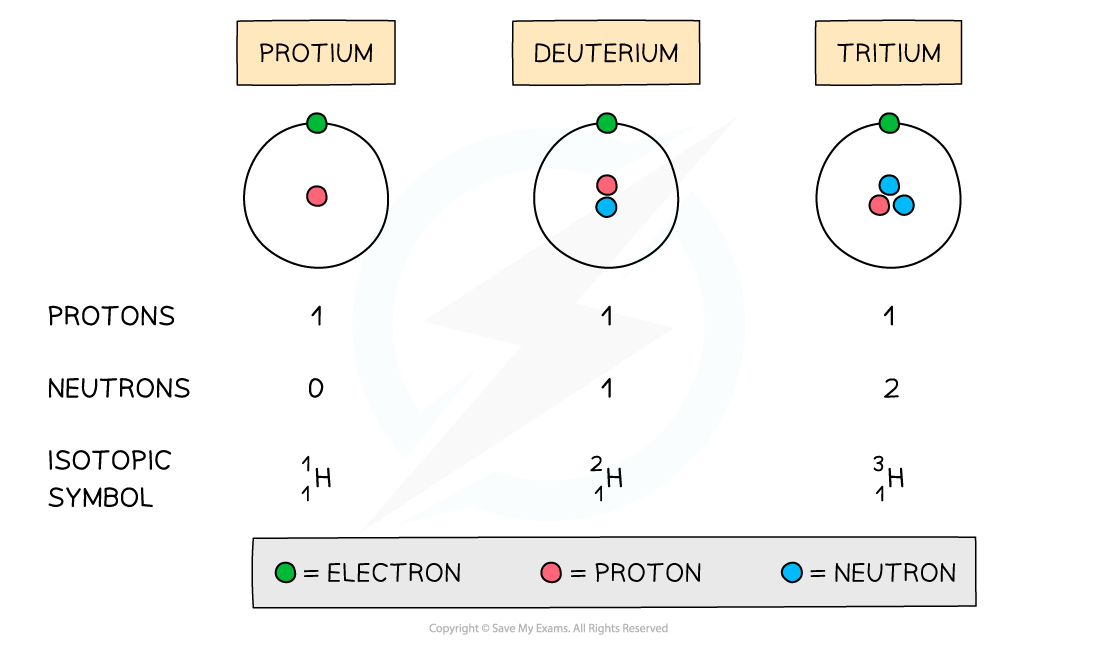The atomic structure and symbols of the three isotopes of hydrogen

### RAM from Mass Spectra

• Isotopes have the same chemical properties but different physical properties

#### Chemical properties

• Isotopes of the same element display the same chemical characteristics
• This is because they have the same number of electrons in their outer shells
• Electrons take part in chemical reactions and therefore determine the chemistry of an atom

#### Physical properties

• The only difference between isotopes is the number of neutrons
• Since these are neutral subatomic particles, they only add mass to the atom
• As a result of this, isotopes have different physical properties such as small differences in their mass and density
• Isotopes are different atoms of the same element that contain the same number of protons and electrons but a different number of neutrons.
• These are atoms of the same elements but with different mass numbers

• Because of this, the mass of an element is given as relative atomic mass (Ar) by using the average mass of the isotopes
• The relative atomic mass of an element can be calculated by using the relative abundance values
• The relative abundance of an isotope is either given or can be read off the mass spectrum
•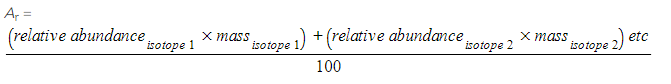#### Worked Example

Calculating relative atomic mass of oxygen
A sample of oxygen contains the following isotopes: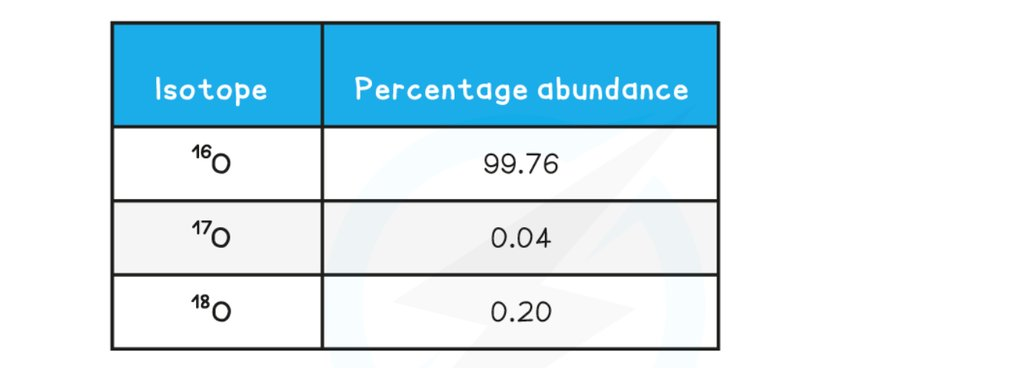What is the relative atomic mass of oxygen in this sample, to 2dp?

1. 16.00
2. 17.18
3. 16.09
4. 17.00

The correct answer option is 1

•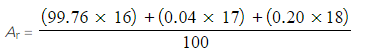• Ar = 16.0044
• Ar = 16.00

#### Worked Example

Calculating relative atomic mass of boron

Calculate the relative atomic mass of boron using its mass spectrum, to 1dp: